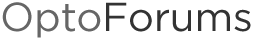# Correct way to scale a resistance input?

Newbie here…For my first learning project, I’ve interfaced a Davis Instruments anemometer and wind vane to, respectively, a SNAP-AIRATE card, and a SNAP AIR40K card. Also A Hydreon RG11 rain sensor configured as a 0.001" per tip tipping bucket rain gauge emulator.

The anny is a magnetic sensor that “closes” once per rev of the wind cups. The direction is a 20K continuous turn pot.

Everything works and I can see the raw values change in a watch window in PAC Control. (The tach module might not be the best for this, as the threshold for the anny is 1 mph = 1 rev in 2.25 seconds. But the module came with the system I bought on ebay, so I thought I’d try it out.)

The question right now is, what’s the proper way to scale/calibrate the 0-20K pot to 0-360 degrees?

Thanks!

Chris

1 Like

There is an example strategy here that shows one way to do to the calibration, using a subroutine. Since you have a linear response, you don’t need to use the more complicated scaling methods that the example shows, and you can stick with just a simple linear calibration.

That example is really more useful when the scaling is non-linear. For something as simple as this, an easy solution would be to set the input scale for the AIR40k module to 0-20000 ohms, and then in one of your charts create a loop (with a delay) that converts the input value to degrees. If you can orient the anemometer so that 0 ohm = 0 deg and 19999 ohm = 359.98 deg, it would be nice, but if there is an offset because north is in a direction different than the anemometer is expecting, you could include that as well.

(output in deg) = [(input in ohm) * (360 / 20000)] + (offset in deg)

Note that when using a offset, a check is needed to keep the output under 360 deg

if ((output in deg) > 360) then
(output in deg) = (output in deg) - 360

If it turns out that there is a scaling problem (0 is not the minimum resistance or 20000 is not the maximum resistance), you could include that too:

(output in deg) = [(input in ohm - minimum resistance) * (360 / (maximum resistance - minimum resistance)] + (offset in deg)

Thanks! The non-linear scaling is useful to me as well. I have a thermistor connected to a SNAP AIR40K-4 card I could linearize, as well.

I finally got around to trying this. It works pretty well. I did, however, figure out that the pot isn’t exactly 20k.That was when my calculation showed the wind direction at 368 degrees.

Rather than hassle with disconnecting and measuring the wind vane pot, I added in a Maximum instruction which compared the current raw pot reading to the current maximum. Once the max stopped climbing, I had a new resistance value to use in the calculation.

I suppose I could make the algorithm auto-calibrating by using the max value in the calculation directly, rather than using it to create a correction factor I manually enter into the program.